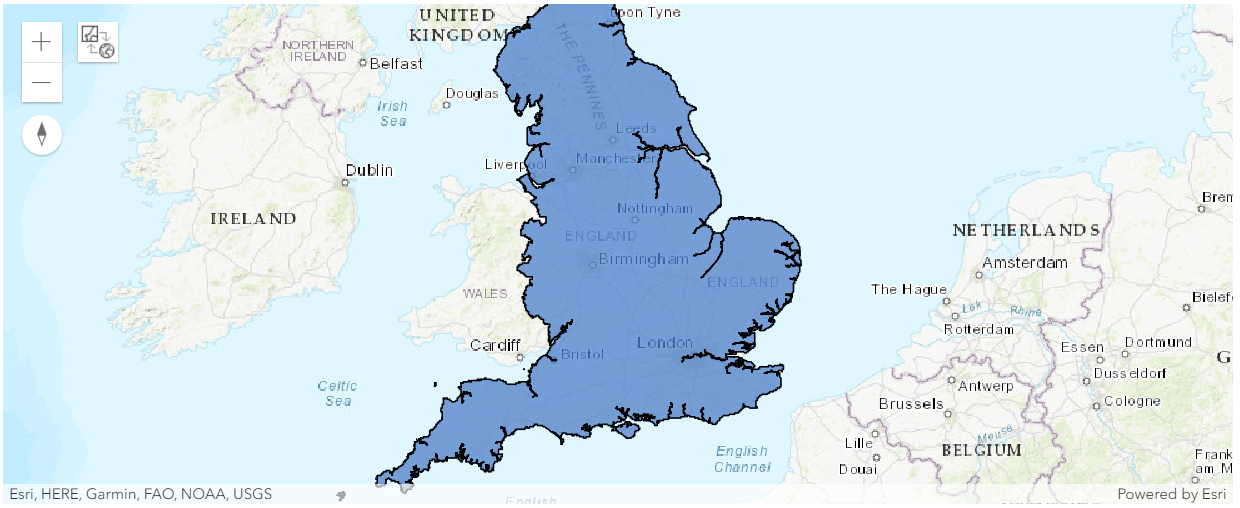ArcGIS Developers
Dashboard

# Temperature forecast using time series data¶

## Introduction ¶

Weather forecasting has been a significant area for application of advanced deep learning and machine learning methodologies over traditional methods to improve weather prediction. These new methods are appropriate for processing large chunks of data where massive quantity of historic weather datasets could be utilized for forecasting. This sample showcases two autoregressive methods: one using a deep learning and another using a machine learning framework to predict temperature of England.

Historic temperature data from various weather stations across England is collected from here. The data consists of daily temperature measurements ranging from February 2005 till September 2019 which are auto regressed to predict daily temperature for each of the identified stations for October 2019. The forecasted temperature obtained for the stations is then spatially interpolated using ArcGIS spatial interpolation tool to produce a temperature prediction surface for the entire country. Here is a schematic flow chart of the operation:## Prerequisites¶

Some required libraries for this sample are NumPy for processing arrays, pandas for operating with DataFrame, ArcGIS for geoprocessing, scikit-learn=0.22.1 for machine learning, tensorflow=2.0.0 and keras=2.2.4 for deep learning.

## Necessary Imports ¶

In :
```%matplotlib inline
import matplotlib.pyplot as plt

import numpy as np
import pandas as pd
import math
from datetime import datetime as dt
from IPython.display import Image, HTML

from sklearn.svm import SVR
from sklearn.preprocessing import MinMaxScaler
from sklearn.metrics import mean_squared_error, r2_score

from tensorflow.keras.models import Sequential
from tensorflow.keras.layers import LSTM, Dense, Activation, Dropout
import tensorflow.keras.backend as K

from arcgis.gis import GIS
from arcgis.features import SpatialDataFrame
from arcgis.features.analysis import interpolate_points
```

## Connect to your GIS ¶

In :
```gis = GIS('home')
```

## Obtain and Visualize Data for Analysis¶

The primary data used for this sample is as follows:

### Data 1— England Boundary ¶

First the boundary of England shapefile is accessed. This will be used to interpolate temperature within this particular area.

In :
```# Get the feature layer
england_boundary_layer = england_border.layers
```
In :
```# Plot England boundary
england_map = gis.map('England', zoomlevel=6)
england_map
```### Data 2 — England Weather Stations ¶

There are several weather stations in England that record a variety of weather data. Here 29 weather stations are strategically selected such that they are well distributed across England and can be used to forecast temperature which will cover the entire country. These include stations located at prominent English cities such as London, Birmingham, Cardiff, Exeter, Nottingham, Plymouth and others, as shown in the map below.

In :
```england_weather_stations_layer = england_weather_stations.layers
```
In :
```# England weather stations
england_weather_stations_map = gis.map('England', zoomlevel=6)